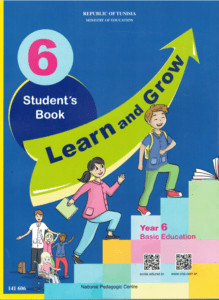# 6 Form Basic Education – New

The official book and audio files used in the 6th form basic education in Tunisian schools according to the New official programme of the school year 2022-2023.

Student bookFile format: PDF

File Size: 15.8 MB

Audio Files

Please find the listening passages. If you want to download an audio file, please click on the 3 dots. U= Unit / L= Lesson

## UNIT 1: Entertaining Myself

1. Lesson 1 : Let’s have fun / Let's get started

2. Lesson 1 : Let’s have fun / Let's pronouce

3. Lesson 2 : My free time activities / Let's read

4. Lesson 2 : My free time activities / Let's pronouce

5. Lesson 3 : A day out / Let’s listen

6. Lesson 3 : A day out / Let's pronouce

7. Lesson 4 : Let’s go on a picnic / Let's read

8. Lesson 4 : Let’s go on a picnic / Let's pronouce

9. Lesson 5 : Pass the ball / Let's read

10. Lesson 5 : Pass the ball / Let's pronouce

## UNIT 2 : keeping fit

1. Lesson 1 : I’m healthy , I’m happy / Let's pronouce

2. Lesson 2 : Eat well , live well / Let's listen

3. Lesson 2 : Eat well , live well / let's pronouce

4. Lesson 3 : I’m healthy , I’m fit / Let's read

5. Lesson 3 : I’m healthy , I’m fit / Let's pronouce

6. Lesson 4 : How are you feeling ? / Let's get started

7. Lesson 4 : How are you feeling ? / Let's read

8. Lesson 4 : How are you feeling ? / Let's pronouce

9. Lesson 5 : How to stay fit ? / Let's pronouce

## UNIT 3 : Having fun in the seasons

1. Lesson 1 : The four seasons / Let's get started

2. Lesson 1 : The four seasons / Let's pronounce

3. Lesson 2 : Having fun in spring / Let's read

4. Lesson 2 : Having fun in spring / Let's pronounce

5. Lesson 3 : Having fun in summer / Let's read

6. Lesson 3 : Having fun in summer / Let's pronounce

7. Lesson 4 : Having fun in autumn / Let's read

8. Lesson 4 : Having fun in autumn / Let's pronounce

9. Lesson 5: Having fun in winter / Let's read

10. Lesson 5: Having fun in winter / Let's pronounce 1

11. Lesson 5: Having fun in winter / Let's pronounce 2

## UNIT 4 : Caring

1. Lesson 1 : Let’s help each other / Let's listen

2. Lesson 1 : Let’s help each other / Let's pronounce

3. Lesson 2 : Sharing and caring / Let's read

4. Lesson 2 : Sharing and caring / Let's pronounce

5. Lesson3: We are stronger together / Let's read

6. Lesson3: We are stronger together / Let's pronounce

7. Lesson 4: Caring for my school / Let's listen

8. Lesson 4: Caring for my school / Let's pronounce

9. Lesson 5: Caring for my pet / Let's get started

10. Lesson 5: Caring for my pet / Let's read

11. Lesson 5: Caring for my pet / Let's pronounce

## UNIT 5 : Celebrations

1. Lesson 1: Children’s Day / Let's speak

2. Lesson 1: Children’s Day / Let's pronounce

3. Lesson 2 : Mother’s Day / Let's listen

4. Lesson 2 : Mother’s Day / Let's pronounce

5. Lesson 3 : Happy new year / Let's read

6. Lesson 3 : Happy new year / Let's pronounce

7. Lesson 4 : Teacher’s Day / Let's listen

8. Lesson 4: Teacher’s Day / Let's pronounce

9. Lesson 5 : End of school year party / Let's read

10. Lesson 5 : End of school year party / Let's pronounce

## UNIT 6 : Going shopping

1. Lesson1: Shops in my town / Let's pronounce

2. Lesson2: At the market / Let's listen

3. Lesson2: At the market / Let's pronounce

4. Lesson 3: At the toy shop / Let's read

5. Lesson 3: At the toy shop / Let's pronounce

6. Lesson 4 : At the clothes shop / Let's get started

7. Lesson 4 : At the clothes shop / Let's listen

8. Lesson 4 : At the clothes shop / Let's pronounce

9. Lesson 5 : At the mall / Let's read

10. Lesson 5 : At the mall / Let's pronounce

11. Lesson 5 : At the mall / Progress check 6

## UNIT 7 : Around the world

1. Lesson1 : Let ‘s travel to…/ Let's get started

2. Lesson1 : Let ‘s travel to…/ Let's pronounce

3. Lesson2 : Greeting around the world / Let's read

4. Lesson2 : Greeting around the world / Let’s pronounce

5. Lesson 3 : I prefer to travel by …/ Let's listen

6. Lesson 3 : I prefer to travel by …/ Let's pronounce

7. Lesson 4 : Monuments in the world / Let's get started

8. Lesson 4 : Monuments in the world / Let's read

9. Lesson 4 : Monuments in the world / Let’s pronounce

10. Lesson 5 : Customs and habits / Let's read

11. Lesson 5 : Customs and habits / Let’s pronounce